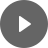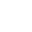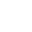4.1 Right-Angled Triangle - StudyNinja
Select Page

HSC Standard Maths Resources

Browse: 1. Home  »  4. Trigonometry  »  4.1 Right-Angled Triangle

4.1 Right-Angled Triangle

These are the easiest forms of question that can be asked in the exam and involves all the basic stuff of right-angled triangle. It is important to get the following basics under control.

Right-angled triangle is one that has one angle of 90o. Each of its edges has certain names. See below:Edge opposite right angle is called ‘Hypotenuse’. Angle of consideration in this triangle is ϴ and the edge next to it (other than hypotenuse) is called ‘Adjacent’, while the edge opposite the angle is called ‘Opposite’.You may be given an angle and a side of a triangle and may be asked to work out another side using the formula above, or you may be given two sides and may be asked to work out an angle.

Get access to 20 Mock Exams with over 700 exam-style questions for HSC Standard Maths.

Example 1

Calculate the value of x for the following triangles:Example 2

Calculate the value of ϴ for the following triangles:You might find following videos helpful related to this section:4A Trigonometric Ratios (1 of 3)

by Mr Bodgers (click to view channel)

Explains how to label the sides of a triangle. Also explains how to convert between angles into degree, minutes and seconds.4A Trigonometric Ratios (2 of 3)

by Mr Bodgers (click to view channel)

Explains how to calculate sides on a right-angled triangle using trigonometric ratios. Makes reference to the SOHCAHTOA acronym.4A Trigonometric Ratios (3 of 3)

by Mr Bodgers (click to view channel)

Explains how to calculate an angle using trigonometric ratios on a right-angled triangle. Refers to the SOHCAHTOA acronym.4B Angles of Elevation and Depression (1 of 2)

by Mr Bodgers (click to view channel)

Explains the difference between angles of elevation and depression and then completes an example involving an angle of elevation.4B Angles of Elevation and Depression (2 of 2)

by Mr Bodgers (click to view channel)

Explains how to solve a problem involving an angle of depression.There are broadly 3 types of questions you can expect in exam:

Study notes of this section and other resources can be accessed here:

Browse: 1. Home  »  4. Trigonometry  »  4.1 Right-Angled Triangle